# Chapter 7 Chemical Reactions 7 1 Describing Reactions

• Slides: 74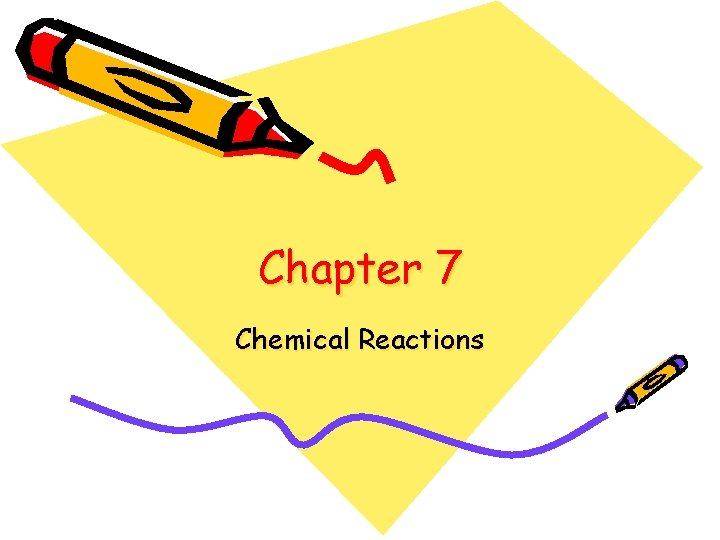Chapter 7 Chemical Reactions7 -1 Describing Reactions • In a chemical reaction, the substances that undergo change are called reactants • The new substances formed as a result of that change are called products • Reactants Products • Ex. Carbon + oxygen carbon dioxide • Word equation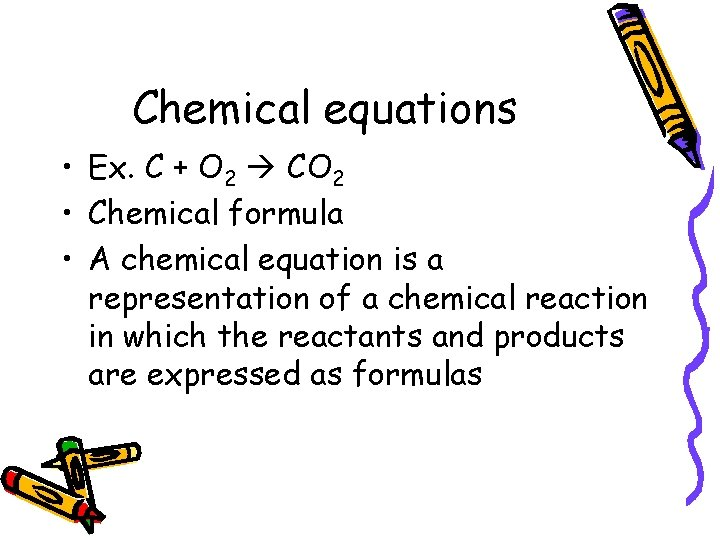Chemical equations • Ex. C + O 2 CO 2 • Chemical formula • A chemical equation is a representation of a chemical reaction in which the reactants and products are expressed as formulasConservation of Mass • During chemical reactions, the mass of the products is always equal to the mass of the reactants. • Established by Antoine Lavoisier and is known as the law of conservation of mass • The law of conservation of mass states that mass is neither created nor destroyed in a chemical reactionHow is mass conserved in chemical change? Activity • Follow the directions on page 191 in your book • Fill out following information • Mass of the bag and the water=______ • Mass of a square piece of paper with sides 10 cm_________ • Mass of the tablet and the paper together______ • Mass of just the tablet________ • The combined masses of the bag, the water, and the tablet______ • Mass of the bag and its contents_______Activity questions 1. How do you know whether a chemical change took place?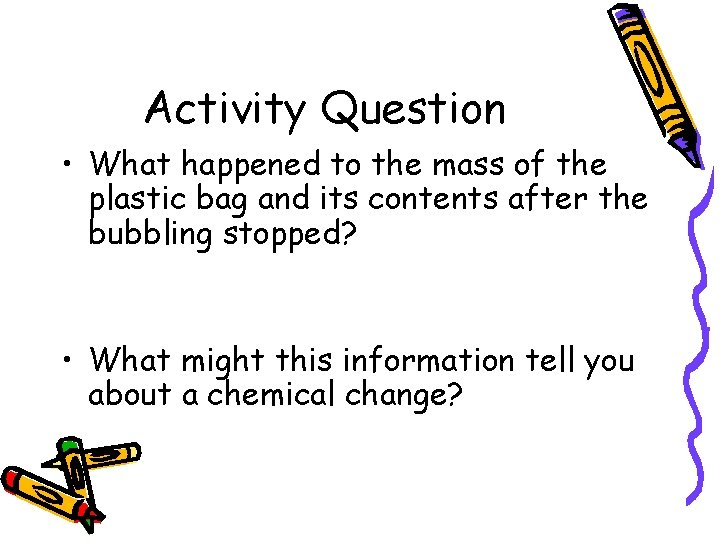Activity Question • What happened to the mass of the plastic bag and its contents after the bubbling stopped? • What might this information tell you about a chemical change?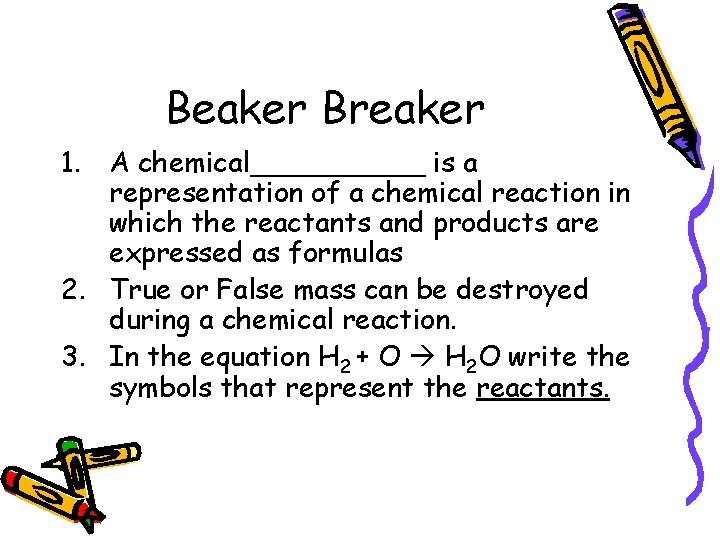Beaker Breaker 1. A chemical_____ is a representation of a chemical reaction in which the reactants and products are expressed as formulas 2. True or False mass can be destroyed during a chemical reaction. 3. In the equation H 2 + O H 2 O write the symbols that represent the reactants.Balancing Equations 7 -1 Continued • In order to show that mass is conserved during a reaction, a chemical equation must be balanced • You can balance a chemical equation by changing the coefficients, the numbers that appear before the formulas • As you balance equations, you should never change the subscripts in a formulaSteps to balancing equations 1. Count the number of atoms of each element on each side of the equation 2. Ex: N 2 H 4 + O 2 N 2 + H 2 O 3. Left side has 2 nitrogen, four hydrogen, and 2 oxygen atoms 4. The right side has 2 nitrogen, 2 hydrogen, and 1 oxygen atom 5. The hydrogen and oxygen atoms need to be balanced.Steps to balancing equations 6. Change one or more coefficients until the equation is balanced _1_N 2 H 4 + _1_O 2 _1_N 2 + _2_H 2 O • • The equation is now balanced Each side has two nitrogen, 2 oxygen, and 4 hydrogen atoms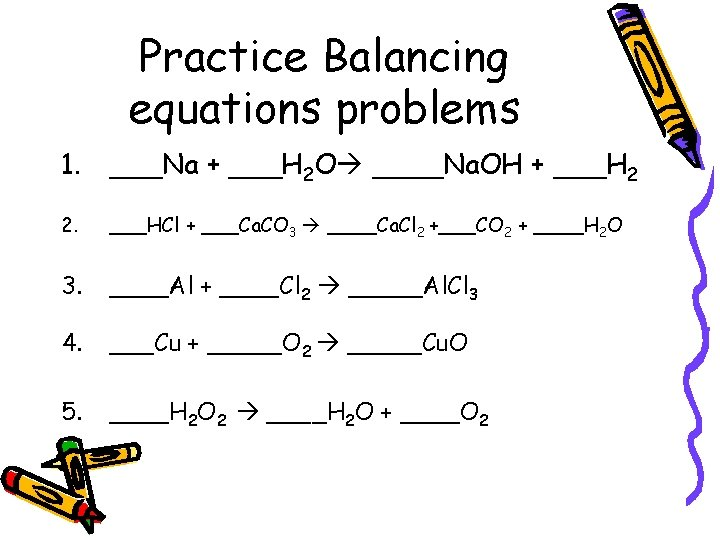Practice Balancing equations problems 1. ___Na + ___H 2 O ____Na. OH + ___H 2 2. ___HCl + ___Ca. CO 3 ____Ca. Cl 2 +___CO 2 + ____H 2 O 3. ____Al + ____Cl 2 _____Al. Cl 3 4. ___Cu + _____O 2 _____Cu. O 5. ____H 2 O 2 ____H 2 O + ____O 2Beaker Breaker • • • Balance the following equation _____Fe + _______Cl 2 ______Fe. Cl 3 _______K + _______Br 2 ______KBr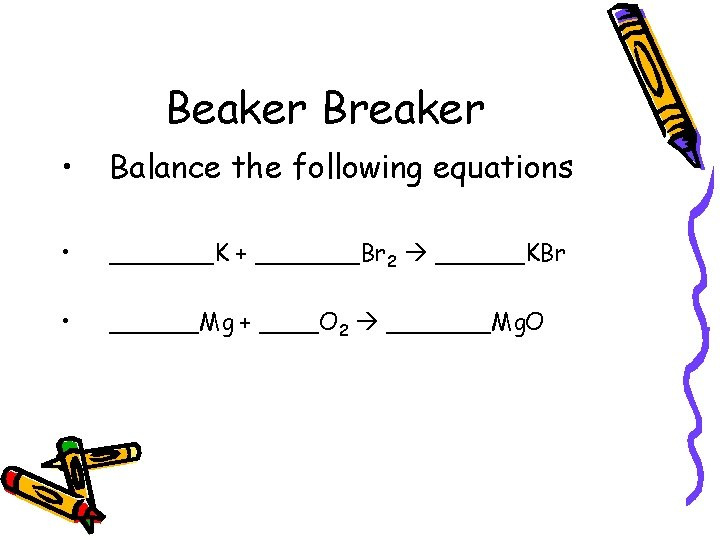Beaker Breaker • Balance the following equations • _______K + _______Br 2 ______KBr • ______Mg + ____O 2 _______Mg. OScientists work with samples large enough for us to SEE and WEIGH on a balance using units of ……. GRAMS.This Creates a Problem…… A pile of atoms big enough for us to see contains billions of atoms. How do we keep track of that many atoms? ? ?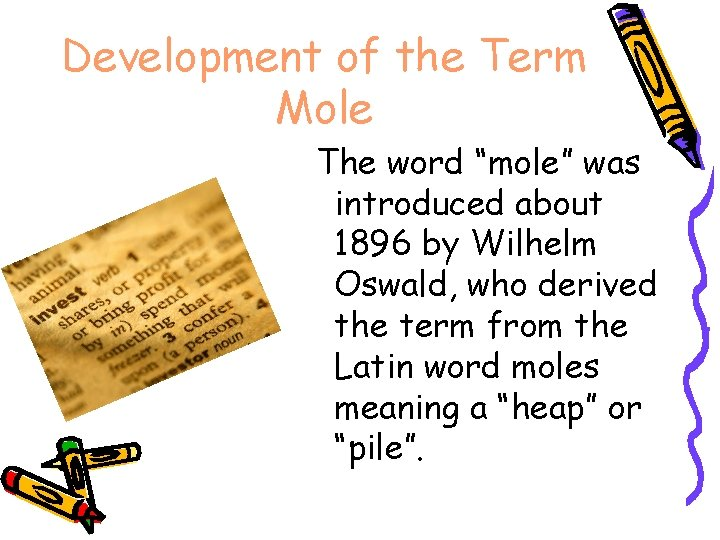Development of the Term Mole The word “mole” was introduced about 1896 by Wilhelm Oswald, who derived the term from the Latin word moles meaning a “heap” or “pile”.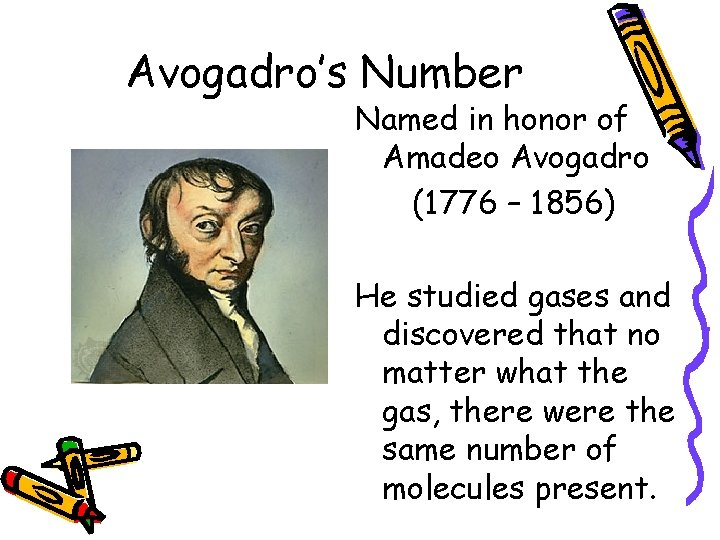Avogadro’s Number Named in honor of Amadeo Avogadro (1776 – 1856) He studied gases and discovered that no matter what the gas, there were the same number of molecules present.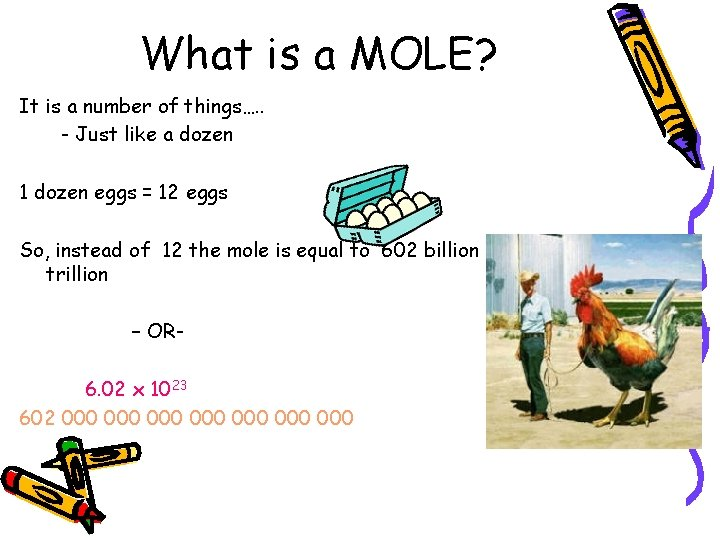What is a MOLE? It is a number of things…. . - Just like a dozen 1 dozen eggs = 12 eggs So, instead of 12 the mole is equal to 602 billion trillion – OR 6. 02 x 1023 602 000 000Just How Big is a Mole? 6. 02 X 1023 Pennies: Would make at least 7 stacks that would reach the moon.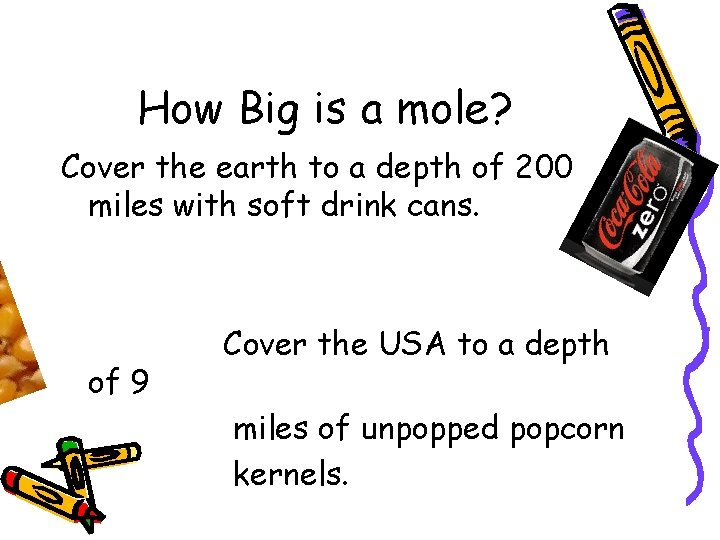How Big is a mole? Cover the earth to a depth of 200 miles with soft drink cans. of 9 Cover the USA to a depth miles of unpopped popcorn kernels.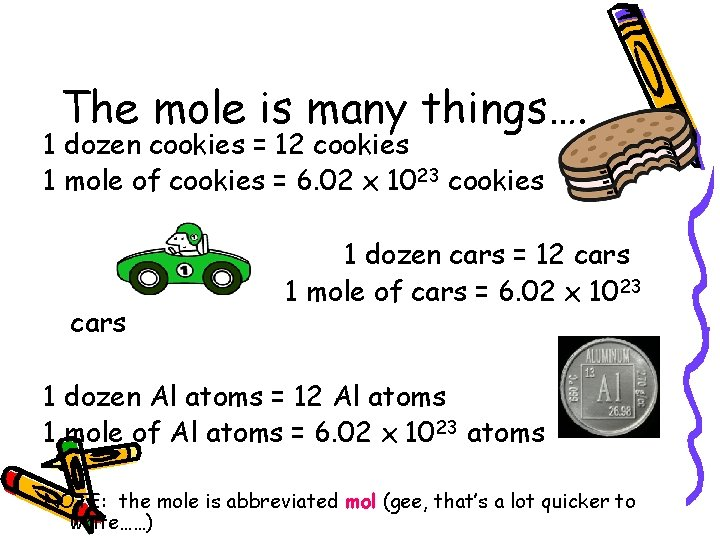The mole is many things…. 1 dozen cookies = 12 cookies 1 mole of cookies = 6. 02 x 1023 cookies cars 1 dozen cars = 12 cars 1 mole of cars = 6. 02 x 10 23 1 dozen Al atoms = 12 Al atoms 1 mole of Al atoms = 6. 02 x 1023 atoms NOTE: the mole is abbreviated mol (gee, that’s a lot quicker to write……)Everybody Dance Now!!!!!!! Moleshake My moleshake brings all the moles to the yard, And they’re like, “Man, Chemistry’s hard” Yeah right, yeah Chemistry’s hard, I can teach you, and I won’t even charge.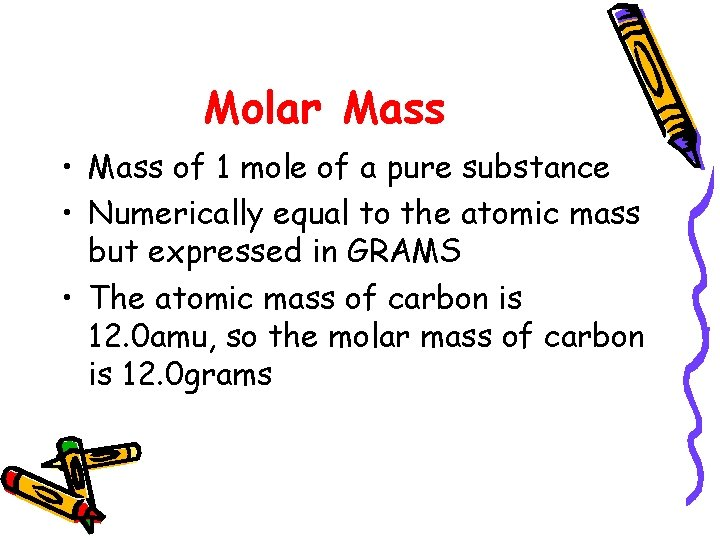Molar Mass • Mass of 1 mole of a pure substance • Numerically equal to the atomic mass but expressed in GRAMS • The atomic mass of carbon is 12. 0 amu, so the molar mass of carbon is 12. 0 grams• What is the molar mass of the following: • Potassium ? • 39. 098 g (or 39. 098 g/mol) Nickel ? • 58. 693 g (or 58. 693 g/mol)• What is the atomic mass of the following: • Potassium ? • 39. 098 amu Nickel ? • 58. 693 amuPractice • 1. 2. 3. 4. What is the molar mass of the following: KCl C 2 H 6 Ca. CO 3 H 2 O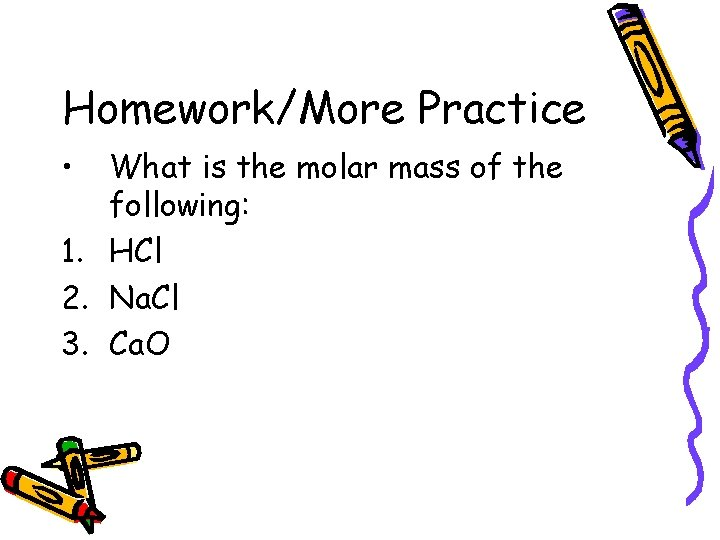Homework/More Practice • What is the molar mass of the following: 1. HCl 2. Na. Cl 3. Ca. OHomework/More Practice • Balance the following ___Na + ____H 2 O ___H 2 + ___Na. OH ___C 2 H 6 + ____O 2 ___CO 2 + ___H 2 O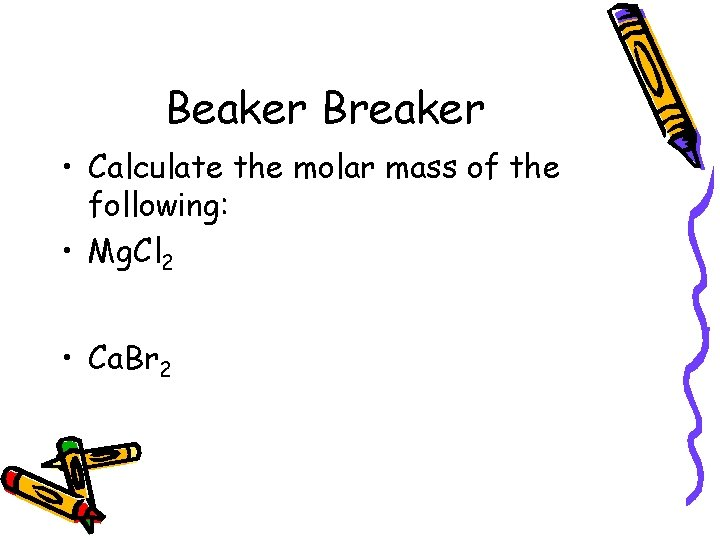Beaker Breaker • Calculate the molar mass of the following: • Mg. Cl 2 • Ca. Br 2Dude!!! Atoms are REALLY small!!! We can’t work with individual atoms or mass individual atoms. BECAUSE……. . We can’t see things that small!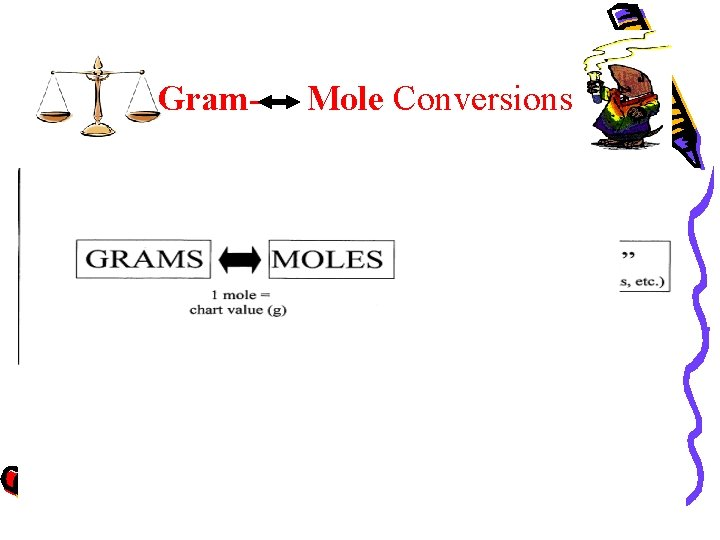Gram- Mole ConversionsHow many grams are needed to have 0. 852 moles gold? • 0. 852 mol Au 1 = 168 g Au 196. 97 g Au 1 mol Au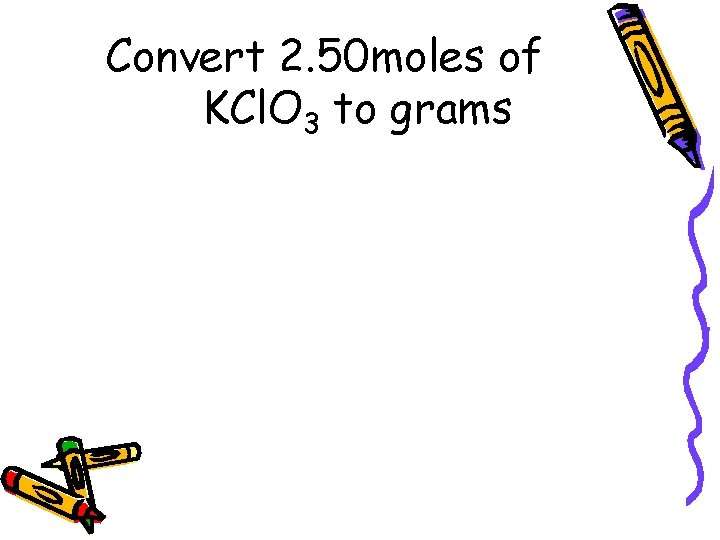Convert 2. 50 moles of KCl. O 3 to grams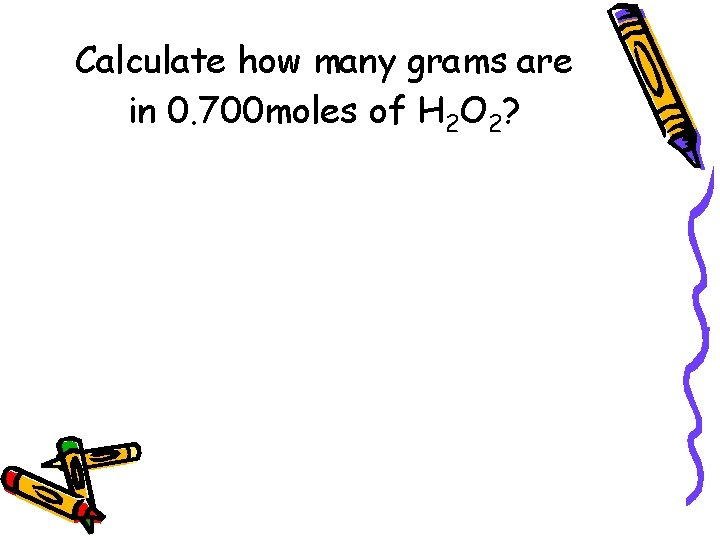Calculate how many grams are in 0. 700 moles of H 2 O 2?How many moles are in 55 g of lead? • 55 g Pb 1 1 mol Pb 207. 2 g Pb • = 0. 26 moles Pb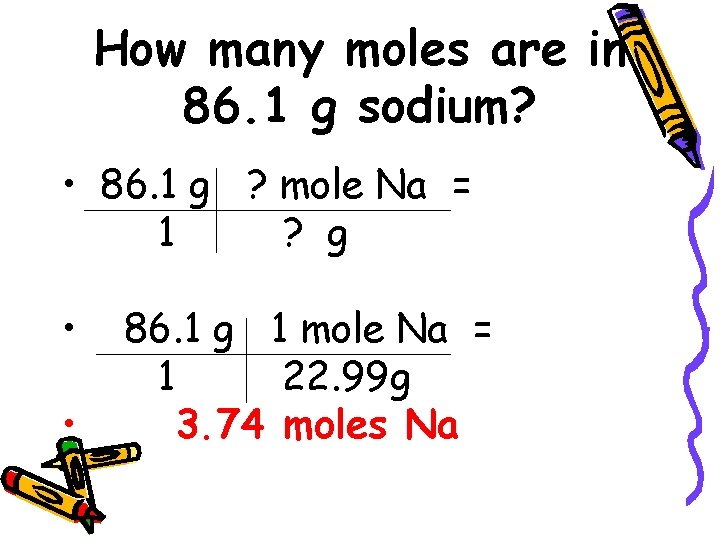How many moles are in 86. 1 g sodium? • 86. 1 g ? mole Na = 1 ? g • • 86. 1 g 1 mole Na = 1 22. 99 g 3. 74 moles NaHow many moles are in 22 grams of copper metal?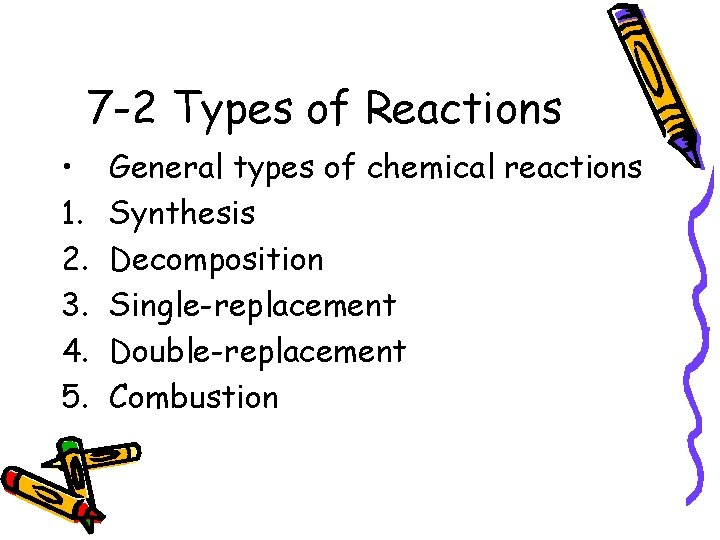7 -2 Types of Reactions • 1. 2. 3. 4. 5. General types of chemical reactions Synthesis Decomposition Single-replacement Double-replacement Combustion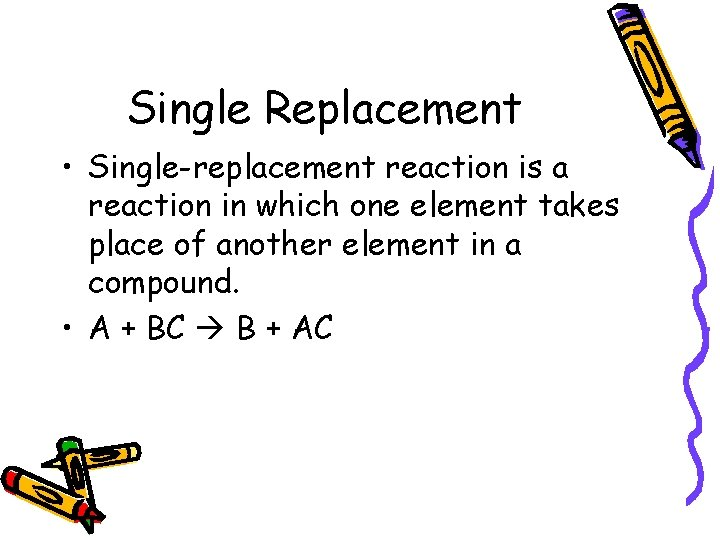Single Replacement • Single-replacement reaction is a reaction in which one element takes place of another element in a compound. • A + BC B + AC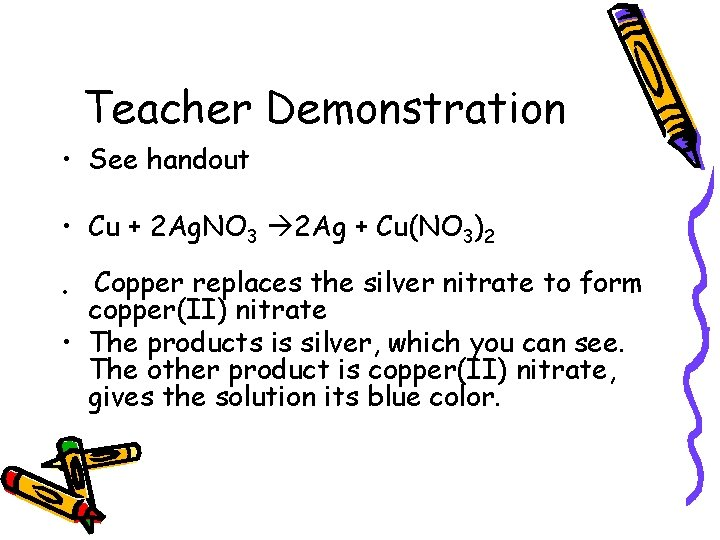Teacher Demonstration • See handout • Cu + 2 Ag. NO 3 2 Ag + Cu(NO 3)2 Copper replaces the silver nitrate to form copper(II) nitrate • The products is silver, which you can see. The other product is copper(II) nitrate, gives the solution its blue color. •7 -2 Continued Reactions as Electron Transfer • The discovery of subatomic particles enabled scientists to classify certain chemical reactions as transfers of electrons between atoms. • A reaction in which electrons are transferred from one reactant to another is called an oxidation-reduction reaction, redox reactionOxidation • Synthesis reactions, in which a metal combines with oxygen, traditionally have been classified as oxidations. • 2 Ca +O 2 2 Ca. O • Ca Ca+2 + 2 e • Calcium loses two electrons • A reactant is oxidized if it loses electronsReduction • As calcium atoms lose electrons during the synthesis of calcium oxide, the oxygen gains electrons • O + 2 e- O 2 • The process in which an element gains electrons during a chemical reaction is called reduction • A reactant is said to be reduced if it gains electrons. • Oxidation and reduction always occur together.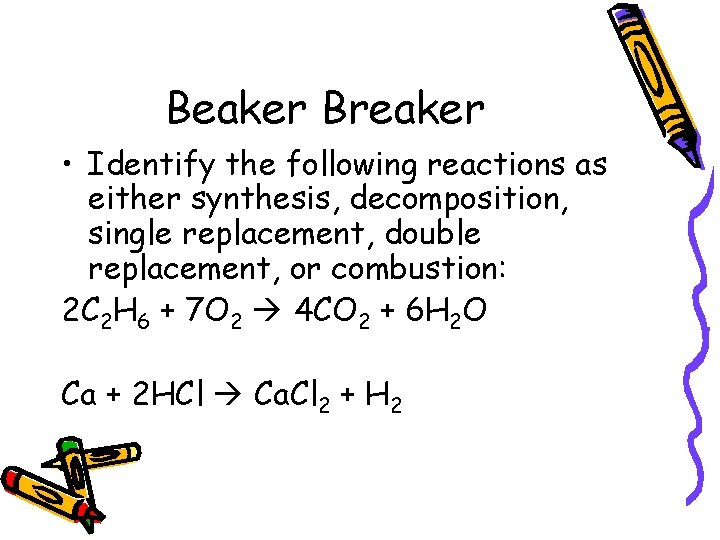Beaker Breaker • Identify the following reactions as either synthesis, decomposition, single replacement, double replacement, or combustion: 2 C 2 H 6 + 7 O 2 4 CO 2 + 6 H 2 O Ca + 2 HCl Ca. Cl 2 + H 2Beaker Breaker Cont. • Write a paragraph explaining why the formation of water can be classified as a synthesis or combustion.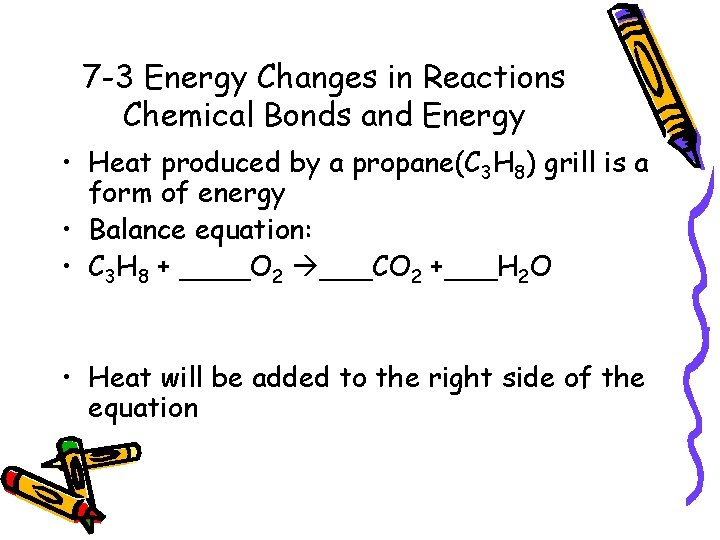7 -3 Energy Changes in Reactions Chemical Bonds and Energy • Heat produced by a propane(C 3 H 8) grill is a form of energy • Balance equation: • C 3 H 8 + ____O 2 ___CO 2 +___H 2 O • Heat will be added to the right side of the equation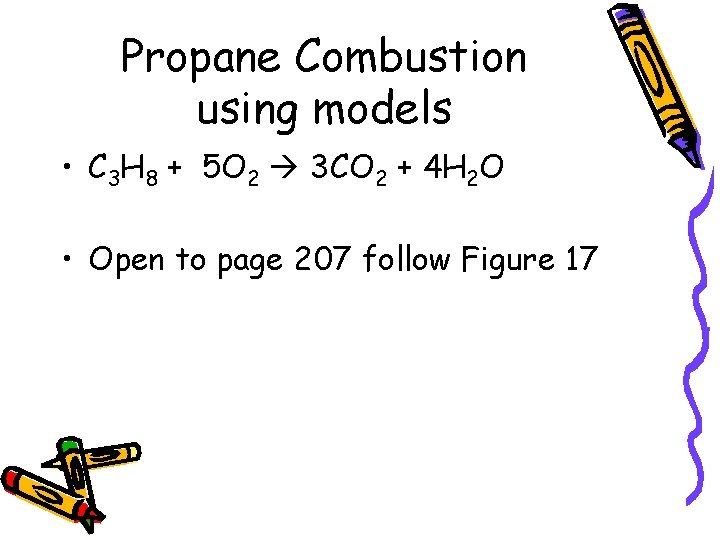Propane Combustion using models • C 3 H 8 + 5 O 2 3 CO 2 + 4 H 2 O • Open to page 207 follow Figure 17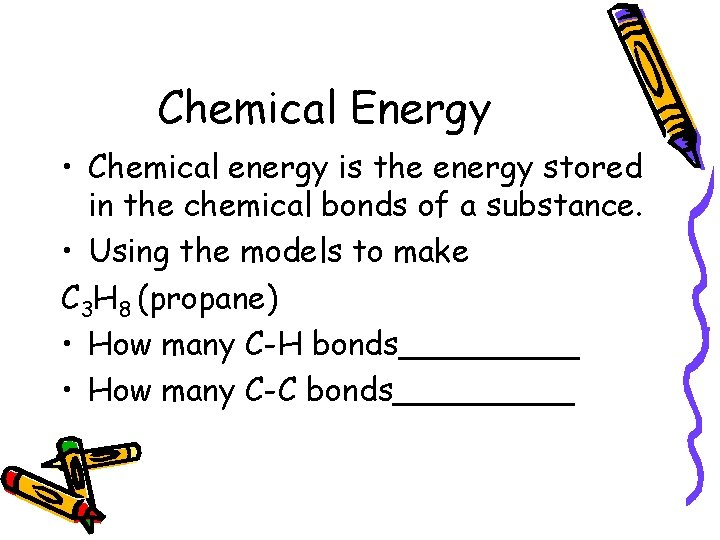Chemical Energy • Chemical energy is the energy stored in the chemical bonds of a substance. • Using the models to make C 3 H 8 (propane) • How many C-H bonds_____ • How many C-C bonds_____Chemical Bonds • Chemical reactions involve the breaking of chemical bonds in the reactants and the formation of chemical bonds in the products. • Each propane molecule reacts with 5 oxygen molecules. • In order for the reaction to occur, the 8 C-H single bonds, 2 C-C single bonds and 5 O=O double bonds must be broken. • Breaking bonds require energy • Propane grills require a igniter to provide enough energy to break the bonds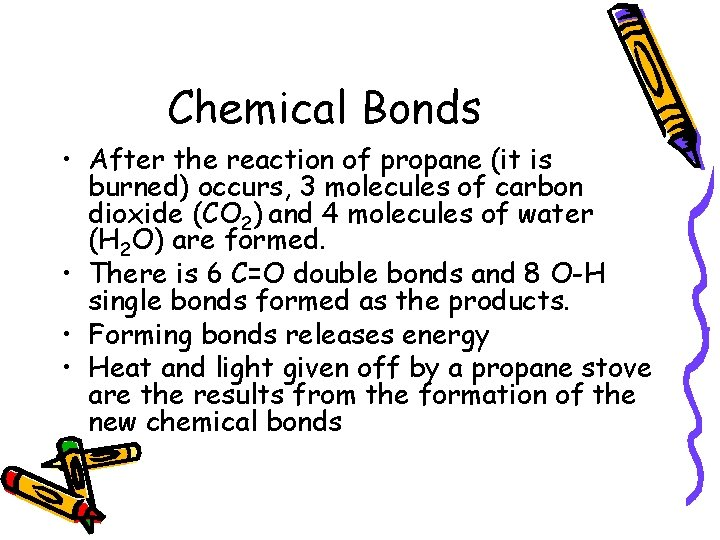Chemical Bonds • After the reaction of propane (it is burned) occurs, 3 molecules of carbon dioxide (CO 2) and 4 molecules of water (H 2 O) are formed. • There is 6 C=O double bonds and 8 O-H single bonds formed as the products. • Forming bonds releases energy • Heat and light given off by a propane stove are the results from the formation of the new chemical bondsHomework • Section 7 -2 Review • #1 , 3, 4, 5Beaker Breaker • Identify the following reactions as either single displacement, double displacement, decomposition, synthesis, or combustion 1. Na. Cl + H 2 SO 4 K 2 SO 4 + H 2 O 2. Ca. O + CO 2 Ca. CO 3 3. Mg. Cl 2 Mg + Cl 2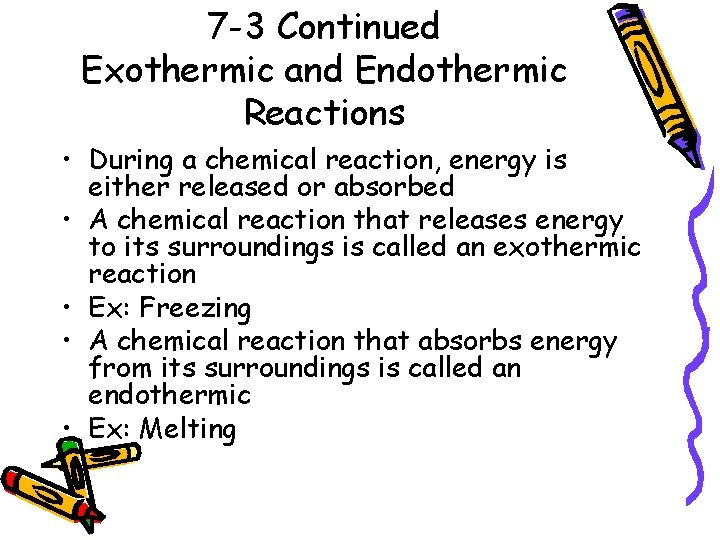7 -3 Continued Exothermic and Endothermic Reactions • During a chemical reaction, energy is either released or absorbed • A chemical reaction that releases energy to its surroundings is called an exothermic reaction • Ex: Freezing • A chemical reaction that absorbs energy from its surroundings is called an endothermic • Ex: MeltingExothermic Reactions • In exothermic reactions, the energy released as the products form is greater than the energy required to break the bonds in the reactants • Example – Combustion • C 3 H 8 + 5 O 2 3 CO 2 + 4 H 2 O + 2220 k. J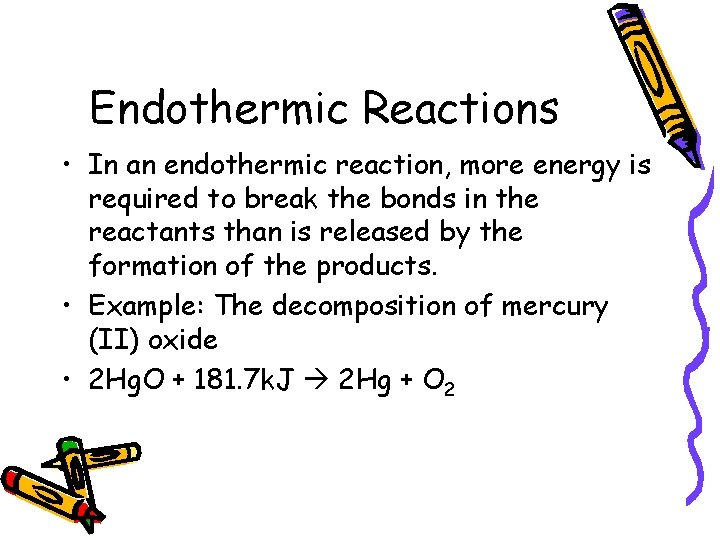Endothermic Reactions • In an endothermic reaction, more energy is required to break the bonds in the reactants than is released by the formation of the products. • Example: The decomposition of mercury (II) oxide • 2 Hg. O + 181. 7 k. J 2 Hg + O 2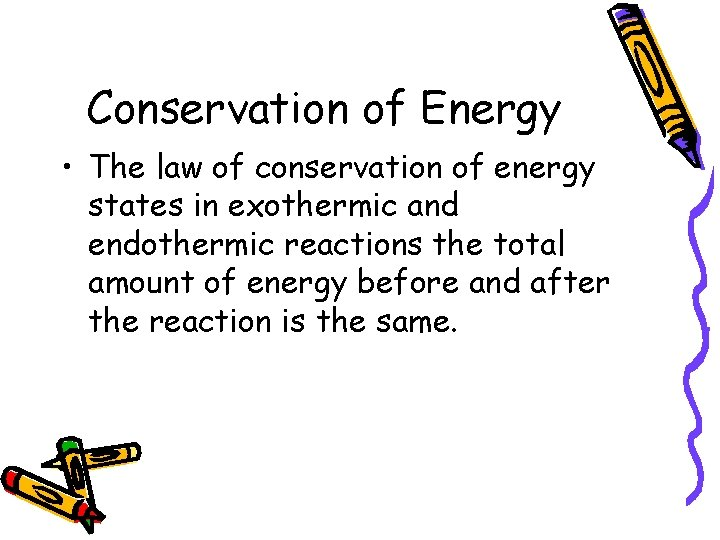Conservation of Energy • The law of conservation of energy states in exothermic and endothermic reactions the total amount of energy before and after the reaction is the same.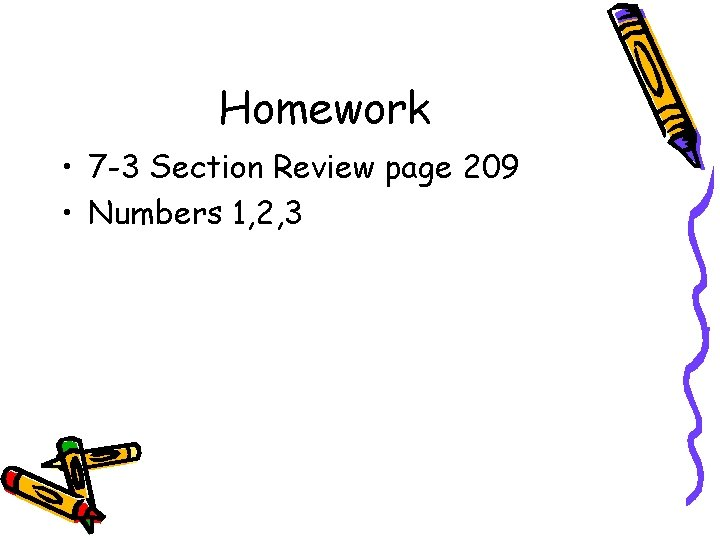Homework • 7 -3 Section Review page 209 • Numbers 1, 2, 3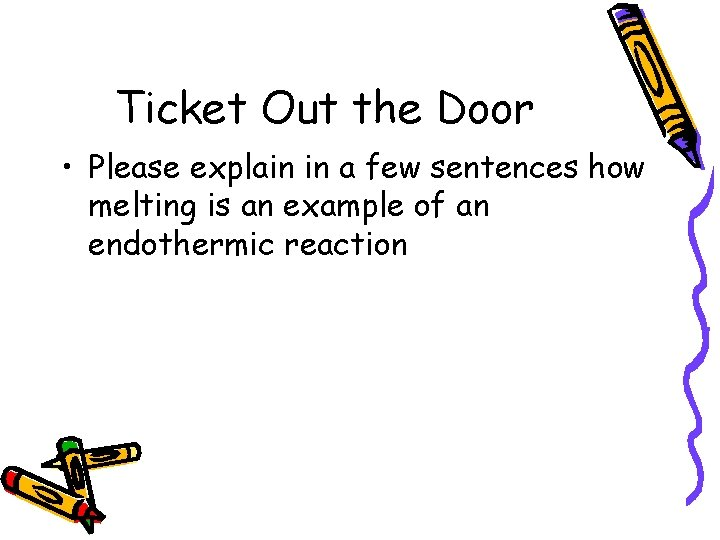Ticket Out the Door • Please explain in a few sentences how melting is an example of an endothermic reaction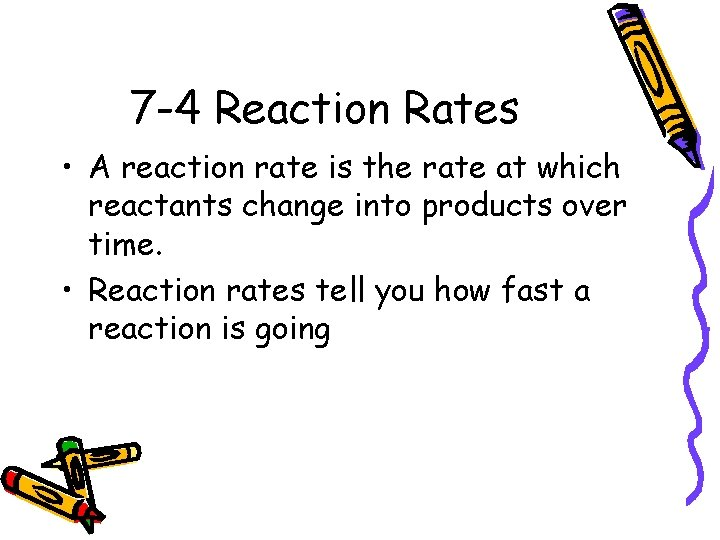7 -4 Reaction Rates • A reaction rate is the rate at which reactants change into products over time. • Reaction rates tell you how fast a reaction is going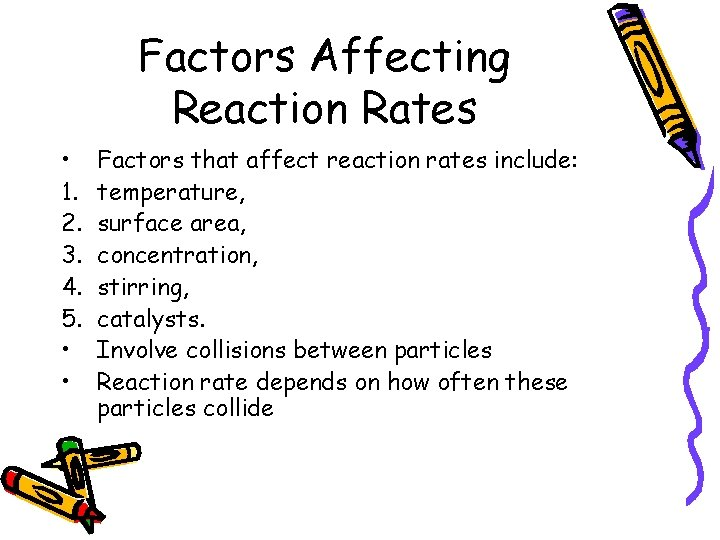Factors Affecting Reaction Rates • 1. 2. 3. 4. 5. • • Factors that affect reaction rates include: temperature, surface area, concentration, stirring, catalysts. Involve collisions between particles Reaction rate depends on how often these particles collide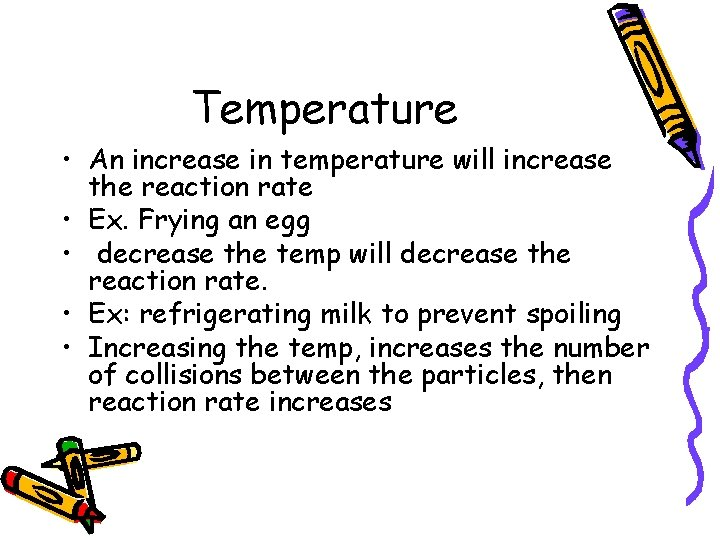Temperature • An increase in temperature will increase the reaction rate • Ex. Frying an egg • decrease the temp will decrease the reaction rate. • Ex: refrigerating milk to prevent spoiling • Increasing the temp, increases the number of collisions between the particles, then reaction rate increasesSurface Area • The smaller the particle size of a given mass, the larger is its surface area. • An increase in surface area increases the exposure of reactants to one another. • The greater this exposure, the more collisions there are that involve reacting particles. • Increasing the surface area of a reactant tends to increase the reaction rate.Beaker Breaker • • • A_____rate is the rate at which reactants change into products over time List two factors that affect reaction rates: An _____ in temperature will_____ the reaction rateStirring • You can increase the exposure of reactants to each other by stirring • Ex: washing machine • Stirring speeds up the reaction. • Collisions between the particles of the reactants are more likely to happenConcentration • Concentration refers to the number of particles in a given volume • Ex: concentrated dyes • The reaction rate is faster • For gases, concentration changes with pressure of a gaseous reactant, the greater is its concentration, and the faster the reaction rate.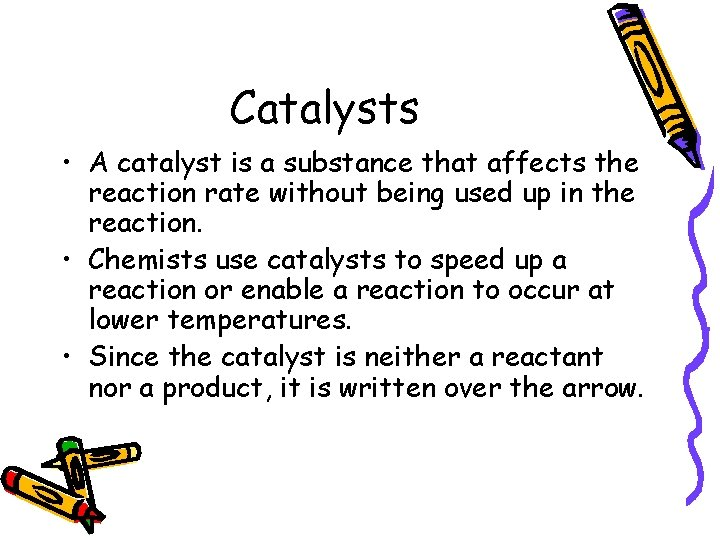Catalysts • A catalyst is a substance that affects the reaction rate without being used up in the reaction. • Chemists use catalysts to speed up a reaction or enable a reaction to occur at lower temperatures. • Since the catalyst is neither a reactant nor a product, it is written over the arrow.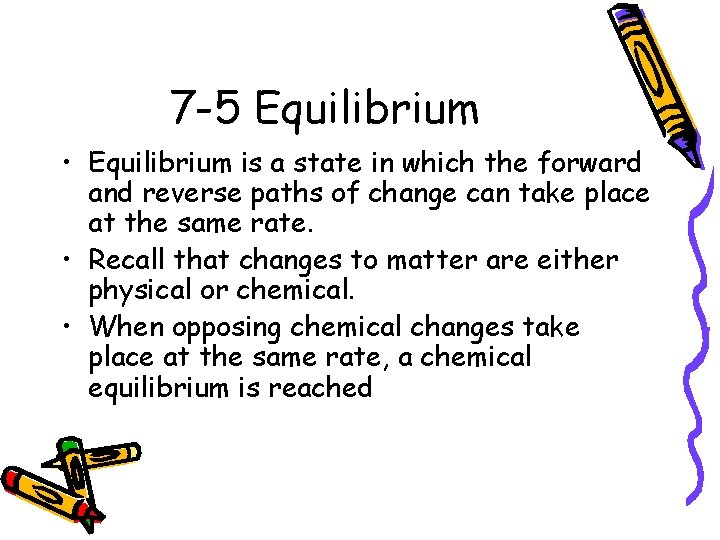7 -5 Equilibrium • Equilibrium is a state in which the forward and reverse paths of change can take place at the same rate. • Recall that changes to matter are either physical or chemical. • When opposing chemical changes take place at the same rate, a chemical equilibrium is reachedPhysical Equilibrium • When a physical change does not go to completion, a physical equilibrium is established between the forward and reverse changes • Ex: H 2 O (l) H 2 O(g) Evaporation and Condensation are both physical changes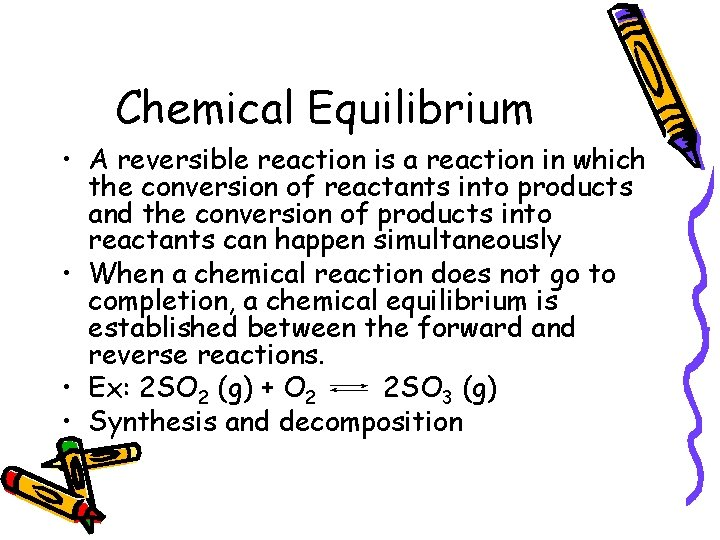Chemical Equilibrium • A reversible reaction is a reaction in which the conversion of reactants into products and the conversion of products into reactants can happen simultaneously • When a chemical reaction does not go to completion, a chemical equilibrium is established between the forward and reverse reactions. • Ex: 2 SO 2 (g) + O 2 2 SO 3 (g) • Synthesis and decomposition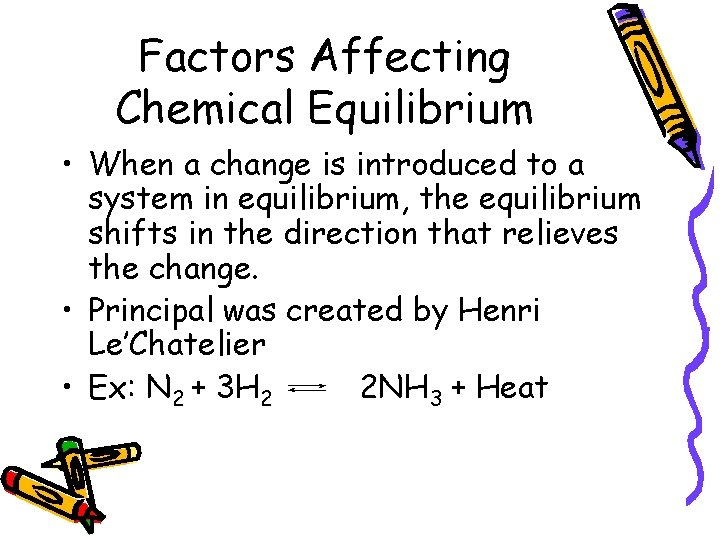Factors Affecting Chemical Equilibrium • When a change is introduced to a system in equilibrium, the equilibrium shifts in the direction that relieves the change. • Principal was created by Henri Le’Chatelier • Ex: N 2 + 3 H 2 2 NH 3 + Heat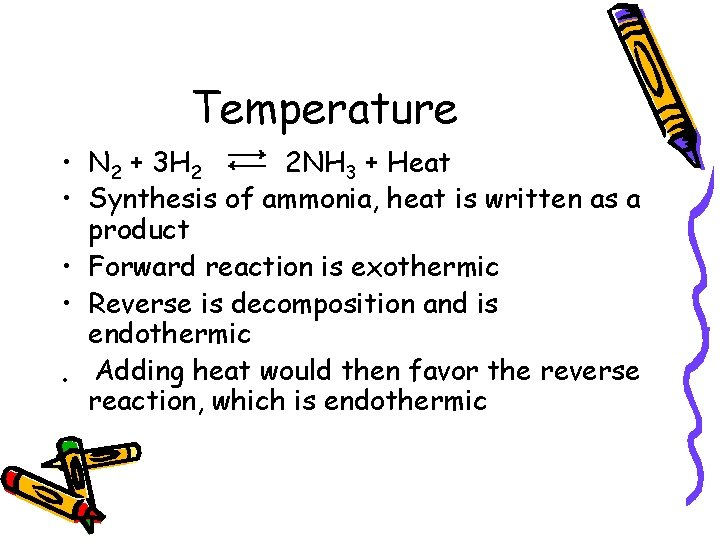Temperature • N 2 + 3 H 2 2 NH 3 + Heat • Synthesis of ammonia, heat is written as a product • Forward reaction is exothermic • Reverse is decomposition and is endothermic • Adding heat would then favor the reverse reaction, which is endothermicPressure • Increase the pressure of the system, equilibrium would shift in the direction that decreases the pressure of the system. • Decreasing the pressure, the system would favor the reaction that produces fewer gas molecules. • Left Side has 4 gas molecules and right side has 2, so equilibrium would shift to the right in order to increase the pressure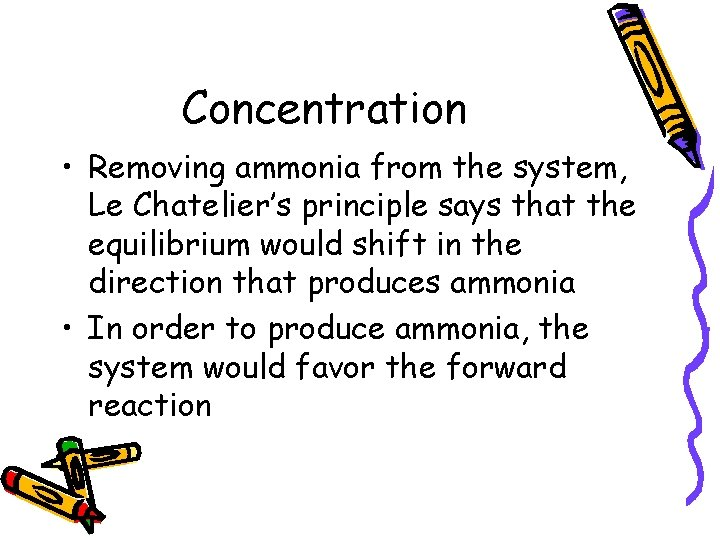Concentration • Removing ammonia from the system, Le Chatelier’s principle says that the equilibrium would shift in the direction that produces ammonia • In order to produce ammonia, the system would favor the forward reaction